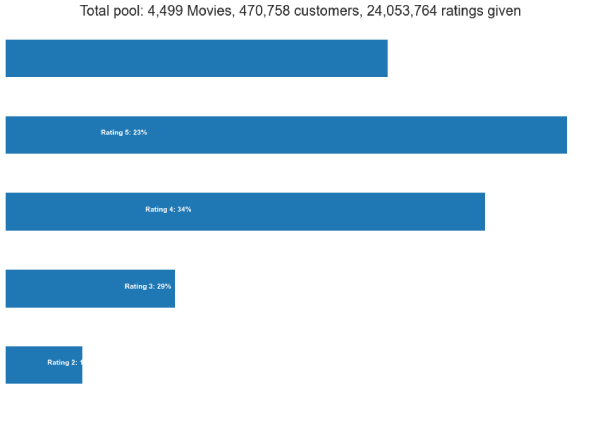• +91-9872993883
• +91-8283824812
• info@ris-ai.com

# Netflix Recommendation System with Python¶

The use of a Recommendation system is to provide users with recommendations based on their search preferences. In this article, I will introduce you to a machine learning project on the Netflix recommendation system with Python.

I will start by importing all the python libraries and the dataset:

In :
import pandas as pd
import numpy as np
import math
import re
from scipy.sparse import csr_matrix
import matplotlib.pyplot as plt
import seaborn as sns
from surprise import Reader, Dataset, SVD
sns.set_style("darkgrid")
# Skip date
df1 = pd.read_csv('Data/combined_data_1.txt', header = None, names = ['Cust_Id', 'Rating'], usecols = [0,1])
df1['Rating'] = df1['Rating'].astype(float)
df = df1
df.index = np.arange(0,len(df))


Now let’s have a look at the spread of the dataset and the ratings of the people on Netflix movies:

In :
p = df.groupby('Rating')['Rating'].agg(['count'])
# get movie count
movie_count = df.isnull().sum()
# get customer count
cust_count = df['Cust_Id'].nunique() - movie_count

# get rating count
rating_count = df['Cust_Id'].count() - movie_count
ax = p.plot(kind = 'barh', legend = False, figsize = (15,10))
plt.title('Total pool: {:,} Movies, {:,} customers, {:,} ratings given'.format(movie_count, cust_count, rating_count), fontsize=20)
plt.axis('off')
for i in range(1,6):
ax.text(p.iloc[i-1]/4, i-1, 'Rating {}: {:.0f}%'.format(i, p.iloc[i-1]*100 / p.sum()), color = 'white', weight = 'bold')We see that the score tends to be relatively positive (> 3). This may be because unhappy customers tend to just leave instead of making an effort to rate. We can keep this in mind that low-rating movies mean they’re generally really bad.

# Data Preparation¶

The Movie ID column is a mess. We’re going to improve this by first creating a NumPy array with the correct length, then adding the entire array as a column in the main dataframe:

In :
df_nan = pd.DataFrame(pd.isnull(df.Rating))
df_nan = df_nan[df_nan['Rating'] == True]
df_nan = df_nan.reset_index()
movie_np = []
movie_id = 1
for i,j in zip(df_nan['index'][1:],df_nan['index'][:-1]):
# numpy approach
temp = np.full((1,i-j-1), movie_id)
movie_np = np.append(movie_np, temp)
movie_id += 1
# Account for last record and corresponding length
# numpy approach
last_record = np.full((1,len(df) - df_nan.iloc[-1, 0] - 1),movie_id)
movie_np = np.append(movie_np, last_record)

print('Movie numpy: {}'.format(movie_np))
print('Length: {}'.format(len(movie_np)))

# remove those Movie ID rows
df = df[pd.notnull(df['Rating'])]

df['Movie_Id'] = movie_np.astype(int)
df['Cust_Id'] = df['Cust_Id'].astype(int)
print('-Dataset examples-')
print(df.iloc[::5000000, :])

Movie numpy: [1.000e+00 1.000e+00 1.000e+00 ... 4.499e+03 4.499e+03 4.499e+03]
Length: 24053764

/.local/lib/python3.7/site-packages/ipykernel_launcher.py:25: SettingWithCopyWarning:
A value is trying to be set on a copy of a slice from a DataFrame.
Try using .loc[row_indexer,col_indexer] = value instead

See the caveats in the documentation: https://pandas.pydata.org/pandas-docs/stable/user_guide/indexing.html#returning-a-view-versus-a-copy

-Dataset examples-
Cust_Id  Rating  Movie_Id
1         1488844     3.0         1
5000996    501954     2.0       996
10001962   404654     5.0      1962
15002876   886608     2.0      2876
20003825  1193835     2.0      3825

/.local/lib/python3.7/site-packages/ipykernel_launcher.py:26: SettingWithCopyWarning:
A value is trying to be set on a copy of a slice from a DataFrame.
Try using .loc[row_indexer,col_indexer] = value instead

See the caveats in the documentation: https://pandas.pydata.org/pandas-docs/stable/user_guide/indexing.html#returning-a-view-versus-a-copy


The dataset is super huge. Therefore, we need to reduce the data volume by improving data quality. We can use these two approaches:

1. Delete the movie with too many reviews (they are relatively unpopular)
2. Remove the customer who gives too less notice (he is relatively less active)
In :
f = ['count','mean']

df_movie_summary = df.groupby('Movie_Id')['Rating'].agg(f)
df_movie_summary.index = df_movie_summary.index.map(int)
movie_benchmark = round(df_movie_summary['count'].quantile(0.7),0)
drop_movie_list = df_movie_summary[df_movie_summary['count'] < movie_benchmark].index
print('Movie minimum times of review: {}'.format(movie_benchmark))

df_cust_summary = df.groupby('Cust_Id')['Rating'].agg(f)
df_cust_summary.index = df_cust_summary.index.map(int)
cust_benchmark = round(df_cust_summary['count'].quantile(0.7),0)
drop_cust_list = df_cust_summary[df_cust_summary['count'] < cust_benchmark].index

print('Original Shape: {}'.format(df.shape))
df = df[~df['Movie_Id'].isin(drop_movie_list)]
df = df[~df['Cust_Id'].isin(drop_cust_list)]
print('After Trim Shape: {}'.format(df.shape))
print('-Data Examples-')
print(df.iloc[::5000000, :])

Movie minimum times of review: 1799.0
Original Shape: (24053764, 3)
After Trim Shape: (17337458, 3)
-Data Examples-
Cust_Id  Rating  Movie_Id
696        712664     5.0         3
6932490   1299309     5.0      1384
13860273   400155     3.0      2660
20766530   466962     4.0      3923


# Netflix Recommendation Algorithm¶

Now let’s build the Netflix recommendation system:

In :
df_p = pd.pivot_table(df,values='Rating',index='Cust_Id',columns='Movie_Id')
df_title = pd.read_csv('Data/movie_titles.csv', encoding = "ISO-8859-1", header = None, names = ['Movie_Id', 'Year', 'Name'])
df_title.set_index('Movie_Id', inplace = True)
def recommend(movie_title, min_count):
print("For movie ({})".format(movie_title))
print("- Top 10 movies recommended based on Pearsons'R correlation - ")
i = int(df_title.index[df_title['Name'] == movie_title])
target = df_p[i]
similar_to_target = df_p.corrwith(target)
corr_target = pd.DataFrame(similar_to_target, columns = ['PearsonR'])
corr_target.dropna(inplace = True)
corr_target = corr_target.sort_values('PearsonR', ascending = False)
corr_target.index = corr_target.index.map(int)
corr_target = corr_target.join(df_title).join(df_movie_summary)[['PearsonR', 'Name', 'count', 'mean']]
print(corr_target[corr_target['count']>min_count][:10].to_string(index=False))

recommend("What the #$*! Do We Know!?", 0)  For movie (What the #$*! Do We Know!?)
- Top 10 movies recommended based on Pearsons'R correlation -
PearsonR                                     Name  count     mean
1.000000               What the #\$*! Do We Know!?  14910 3.189805
0.505500                                Inu-Yasha   1883 4.554434
0.452807 Captain Pantoja and the Special Services   1801 3.417546
0.442354                Without a Trace: Season 1   2124 3.980226
0.384179                     Yu-Gi-Oh!: The Movie   3173 3.331547
0.383959                                 Scorched   2430 2.894239
0.381173  All Creatures Great and Small: Series 1   2327 3.938118
0.381112          As Time Goes By: Series 1 and 2   2249 4.164073
0.373018                         Cowboys & Angels   2368 3.589527
0.371981                           Biggie & Tupac   1866 3.019293